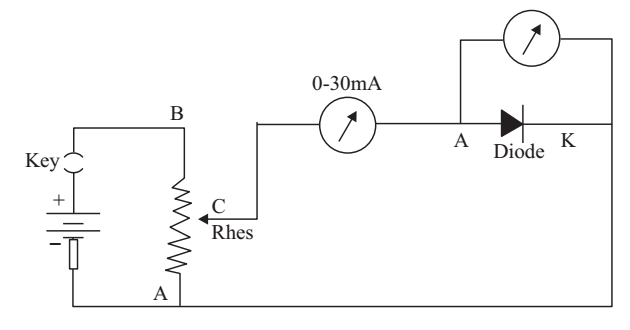# Resistance of Diode Experiment

Experiment: To draw the characteristic curve of a forward biased pn junction diode and to determine the static and dynamic resistance of the diode.

A pn junction diode consists of p-type and n-type materials forming a junction.

In the p-type material there is an impurity of a III group element which gives rise to holes in it. The current flows in it due to motion of these holes. In the n-type material there is an impurity of a V group element which gives rise to free electrons in it. The current flows in it due to motion of these electrons.

Both the materials are electrically neutral. Holes from p-type and electrons from n type, being free, combine with each other at the junction. Due to this combination of holes and electrons, the p-type material develops a negative potential and n-type acquires positive potential. This potential difference across the junction pulls the holes and electrons apart and stops their further combination.

For forward biasing the diode, p-type side of the junction called the ‘Anode’ is connected to the positive pole of the battery and n-type side called the ‘Cathode’ is connected to the negative pole of battery. Under the effect of this external applied potential difference, the holes and electrons are pushed towards each other. When the applied voltage exceeds the contact PD across the junction, they start combining with each other and current starts flowing. It rises rapidly with increase of applied voltage. If the polarity of battery is reversed the holes and electrons are pulled still apart and no current flows in the diode.

In this experiment you have to study, how the current varies with the applied voltage. The current remains zero till the applied voltage approaches contact PD called the ‘knee’ voltage. On increasing the applied voltage beyond this point, the current flowing through the diode increases rapidly. A graph plotted between ‘V and I’ is not a straight line. It is called the characteristics of the diode. In such cases where the (V vs 1) graph is not a straight line, you define two resistances. The static resistance or DC resistance and dynamic resistance or AC resistance. If you take a point P on the curve and note the applied voltage Vp and current Ip corresponding to this point, then the static resistance or Rdc at point P is defined as

R = Vp/Ip

The value of this resistance varies from point to point and is not constant.

The knee voltage of a diode depends on the material used for its fabrication. For Silicon diode its value is 0.7 V and for Germanium diode its value is 0.3 V. The commonly used diodes in the laboratory are OA79 and 1N4007. OA79 is Ge diode which is sealed in a glass tube. 1N4007 is a Silicon diode sealed in plastic casing.

Material Required

A Ge diode OA79, 0-1.5 V voltmeter, 0-30 ma meter, 25 ohm rheostat, 2 V lead accumulator, one-way key, connecting wires.

### How To Perform Experiment

1. Set the zero of both the meters.

2. Record the least count of both the meters.

3. Make the connections as shown in diagram.4. Bring the movable point C of the rheostat nearest to the point A and insert key. Readings in both the meters will be zero. Now move point C slowly towards B so that the reading in the volt meter is on a scale mark and record the readings of mA and volt meter in the observation table.

5. In this manner move point C towards B in small steps and each time take readings of mA and voltmeter. Take the readings in steps of say 0.1 volt till the current passing through the diode is around 25 to 30 mA.

6. Now plot a graph from these readings.

### Sources of Error

1. They may be contact resistances particularly if any connections remains loose.
2. Zero error of the meters may not be accurately eliminated.
3. Starting deflection may be too small and more than 70% of full scale.
4. Each time the pointer of ammeter may not be on a scale mark.
5. Ammeter is measuring current of voltmeter and diode.